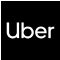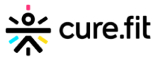New update is available. Click here to update.
Topics

# Merge Intervals

Moderate0/80
Average time to solve is 20m+28 more companies

## Problem statement

You are given N number of intervals, where each interval contains two integers denoting the start time and the end time for the interval.

The task is to merge all the overlapping intervals and return the list of merged intervals sorted by increasing order of their start time.

Two intervals [A,B] and [C,D] are said to be overlapping with each other if there is at least one integer that is covered by both of them.

For example:

``````For the given 5 intervals - [1, 4], [3, 5], [6, 8], [10, 12], [8, 9].

Since intervals [1, 4] and [3, 5] overlap with each other, we will merge them into a single interval as [1, 5].

Similarly, [6, 8] and [8, 9] overlap, merge them into [6,9].

Interval [10, 12] does not overlap with any interval.

Final List after merging overlapping intervals: [1, 5], [6, 9], [10, 12].
``````
Detailed explanation ( Input/output format, Notes, Images )
Constraints:
``````1 <= N <= 10^5
0 <= START, FINISH <= 10^9

Time Limit: 1sec
``````
Sample Input 1:
``````2
1 3
3 5
``````
Sample Output 1:
``````1 5
``````
Explanation for Sample Input 1
``````Since these two intervals overlap at point 3 so we merge them and the new interval becomes (1,5).
``````
Sample Input 2:
``````5
1 3 6 8 10
4 5 8 9 12
``````
Sample Output 2:
``````1 5
6 9
10 12
``````Console